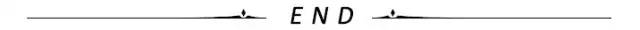# 避免这7个误区，才能让【宏】削铁如泥

10/16 08:14ID：技术让梦想更伟大

## 语法错误

``#define twice(x) (2*(x))#define call_with_1(x) x(1)call_with_1 (twice)//x=1 → twice(1) → (2*(1))``

``#define strange(file) fprintf (file, "%s %d",…strange(stderr) p, 35)     → fprintf (stderr, "%s %d", p, 35)``

## 运算符优先级问题

``#define ceil_div(x, y) (x + y - 1) / y``

``a = ceil_div（b＆c，sizeof（int））;``

``a =（b＆c + sizeof（int）-1）/ sizeof（int）;``

``a =（（（b＆c）+ sizeof（int）-1））/ sizeof（int）;``

``＃define ceil_div（x，y）（（x）+（y）-1）/（y）``

``sizeof（（1）+（2）-1）/（2）``

``＃define ceil_div（x，y）（（（（x）+（y）-1）/（y））``

## 吞噬分号

``#define SKIP_SPACES(p, limit)  \{ char *lim = (limit);         \  while (p < lim) {            \    if (*p++ != ' ') {         \      p--; break; }}}``

``if (*p != 0)  SKIP_SPACES (p, lim);else …``

``#define SKIP_SPACES(p, limit)     \do { char *lim = (limit);         \     while (p < lim) {            \       if (*p++ != ' ') {         \         p--; break; }}}          \while (0)``

`SKIP_SPACES (p, lim);`扩展为

``do {…} while (0);``

## 重复调用

``#define min(X, Y)  ((X) < (Y) ? (X) : (Y))``

``next = min（x + y，foo（z））;``

``next = ((x + y) < (foo (z)) ? (x + y) : (foo (z)));``

``#define min(X, Y)                \({ typeof (X) x_ = (X);          \   typeof (Y) y_ = (Y);          \   (x_ < y_) ? x_ : y_; })``

“（{{…}）”符号产生一个复合表达式，它的值是其最后一条语句的值。

``//假设foo返回int类型#define min(X, Y)  ((X) < (Y) ? (X) : (Y))…{  int tem = foo (z);  next = min (x + y, tem);}``

## 自引用宏

``#define foo (4 + foo)``

1. 对foo的每个引用都将扩展为`（4 + foo）`;

2. 然后将对其进行重新扫描，并将其扩展为`（4 +（4 + foo））`;

3. 以此类推，直到计算机内存耗尽。

``#define EPERM EPERM``

``#define x (4 + y)#define y (2 * x)``

``x→（4 + y）     →（4 +（2 * x））y→（2 * x）     →（2 *（4 + y））``

## 参数预扫描处理

### 调用其他可进行字符串化或连接的宏的宏

``#define AFTERX(x) X_ ## x#define XAFTERX(x) AFTERX(x)#define TABLESIZE 1024#define BUFSIZE TABLESIZE``

### 参数中使用的宏，其扩展名包含未屏蔽的逗号。

``#define foo  a,b#define bar(x) lose(x)#define lose(x) (1 + (x))``

``#define foo (a,b)or#define bar(x) lose((x))``

## 参数中的换行符

``#define ignore_second_arg(a,b,c) a; cignore_second_arg (foo (),                   ignored (),                   syntax error);``

`Syntax error on tokens`触发的语法错误会导致错误消息引用第三行（ignore_second_arg行），即使有问题的代码来自第五行。

### 参考资料：

http://gcc.gnu.org/onlinedocs/cpp/Macros.html推荐阅读：

``````

嵌入式编程专辑

Linux 学习专辑

C/C++编程专辑

Qt进阶学习专辑

关注

微信公众号『技术让梦想更伟大』，后台回复“m”查看更多内容。长按前往图中包含的公众号关注

``````

1
2 收藏

### 作者的其它热门文章0 评论
2 收藏
1